Thermodynamics and Propulsion

# 6.1 Limitations on the Work that Can be Supplied by a Heat Engine

The second law enables us to make powerful and general statements concerning the maximum work that can be derived from any heat engine which operates in a cycle. To illustrate these ideas, we use a Carnot cycle which is shown schematically in Figure 6.1.The engine operates between two heat reservoirs, exchanging heatwith the high temperature reservoir at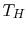and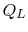with the reservoir at. The entropy changes of the two reservoirs are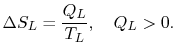The same heat exchanges apply to the system, but with opposite signs: the heat received from the high temperature source is positive, and conversely. Denoting the heat transferred to the engines by subscript'',The total entropy change during any operation of the engine isFor a cyclic process, the third of these ()is zero, and thus (remembering that),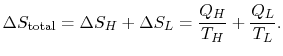(6..1)

For the engine we can write the first law asOr,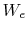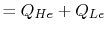Hence, using (6.1)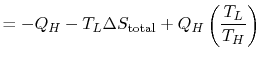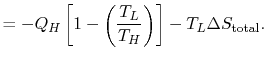The work of the engine can be expressed in terms of the heat received by the engine asThe upper limit of work that can be done occurs during a reversible cycle, for which the total entropy change (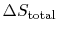) is zero. In this situation: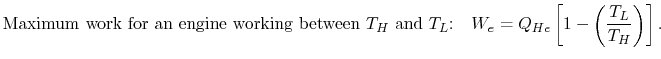Also, for a reversible cycle of the engine,These constraints apply to all reversible heat engines operating between fixed temperatures. The thermal efficiency of the engine is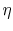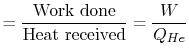The Carnot efficiency is thus the maximum efficiency that can occur in an engine working between two given temperatures.

We can approach this last point in another way. The engine work is given by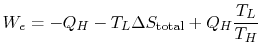or,The total entropy change can be written in terms of the Carnot cycle efficiency and the ratio of the work done to the heat absorbed by the engine. The latter is the efficiency of any cycle we can devise:The second law says that the total entropy change is equal to or greater than zero. This means that the Carnot cycle efficiency is equal to or greater than the efficiency for any other cycle, with the total equality only occurring if.

Muddy Points

So, do we lose the capability to do work when we have an irreversible process and entropy increases? (MP 6.1)

Why do we study cycles starting with the Carnot cycle? Is it because it is easier to work with? (MP 6.2)

UnifiedTP